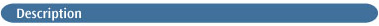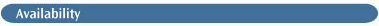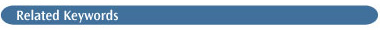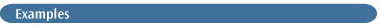# CISCIS(D)The CIS method keyword requests a calculation on excited states using single-excitation CI (CI-Singles) . Chapter 9 of Exploring Chemistry with Electronic Structure Methods  provides a detailed discussion of this method and its uses.

The CIS(D) keyword and option is used to request the related CIS(D) method [426,427]. You can also follow a CIS job with a CIS(D) job to compute the excitation energies for additional states (see the examples).

CIS jobs can include the Density keyword; without options, this keyword causes the population analysis to use the current (CIS) density rather than its default of the Hartree-Fock density. Note that Density cannot be used with CIS(D).

### STATE SELECTION OPTIONS

Singlets
Solve only for singlet excited states. This option only affects calculations on closed-shell systems, for which it is the default.

Triplets
Solve only for triplet excited states. This option only affects calculations on closed-shell systems.

50-50
Solve for half triplet and half singlet states. This option only affects calculations on closed-shell systems.

Root=N
Specifies the "state of interest" for which the generalized density is to be computed. The default is the first excited state (N=1).

NStates=M
Solve for M states (the default is 3). If 50-50 is requested, NStates gives the number of each type of state for which to solve (i.e., the default is 3 singlets and 3 triplets).

Add=N
Read converged states off the checkpoint file and solve for an additional N states. This option implies Read as well. NStates cannot be used with this option.

### DENSITY-RELATED OPTION

AllTransitionDensities

Computes the transition densities between every pair of states.

### PROCEDURE- AND ALGORITHM-RELATED OPTIONS

FC
All frozen core options are available with CIS and CIS(D).

Direct
Forces solution of the CI-Singles equation using AO integrals which are recomputed as needed. CIS=Direct should be used only when the approximately 4O2N2 words of disk required for the default (MO) algorithm are not available, or for larger calculations (over 200 basis functions).

MO
Forces solution of the CI-Singles equations using transformed two-electron integrals. This is the default algorithm in Gaussian 03. The transformation attempts to honor the MaxDisk keyword, thus further moderating the disk requirements.

AO
Forces solution of the CI-Singles equations using the AO integrals, avoiding an integral transformation. The AO basis is seldom an optimal choice, except for small molecules on systems having very limited disk and memory.

Conver=N
Sets the convergence calculations to 10-N on the energy and 10-(N+2) on the wavefunction. The default is N=4 for single points and N=6 for gradients.

Read
Reads initial guesses for the CI-Singles states off the checkpoint file. Note that, unlike for SCF, an initial guess for one basis set cannot be used for a different one.

Restart
Restarts the CI-Singles iterations off the checkpoint file. Also implies SCF=Restart.

RWFRestart
Restarts the CI-Singles iterations off the read-write file. Useful when using non-standard routes to do successive CI-Singles calculations.

EqSolv
Whether to perform equilibrium or non-equilibrium PCM solvation. NonEqSolv is the default.

NoIVOGuess
Forces the use of canonical single excitations for the guess. IVOGuess, which uses improved virtual orbitals, is the default.

### DEBUGGING OPTIONS

ICDiag
Forces in-core full diagonalization of the CI-Singles matrix formed in memory from transformed integrals. This is mainly a debugging option.

MaxDiag=N
Limits the submatrix diagonalized in the Davidson procedure to dimension N. This is mainly a debugging option. MaxDavidson is a synonym for this option.Energies, analytic gradients, and analytic frequencies for CIS, and energies for CIS(D).CIS Output. There are no special features or pitfalls with CI-Singles input. Output from a single point CI-Singles calculation resembles that of a ground-state CI or QCI run. An SCF is followed by the integral transformation and evaluation of the ground-state MP2 energy. Information about the iterative solution of the CI problem comes next; note that at the first iteration, additional initial guesses are made, to ensure that the requested number of excited states are found regardless of symmetry. After the first iteration, one new vector is added to the solution for each state on each iteration.

The change in excitation energy and wavefunction for each state is printed for each iteration (in the #P output):

```Iteration   3 Dimension    27
Root  1 not converged, maximum delta is   0.002428737687607
Root  2 not converged, maximum delta is   0.013107675296678
Root  3 not converged, maximum delta is   0.030654755631835
Excitation Energies [eV] at current iteration:
Root  1 :      3.700631883679401   Change is   -0.001084398684008
Root  2 :      7.841115226789293   Change is   -0.011232152003400
Root  3 :      8.769540624626156   Change is   -0.047396173133051```

The iterative process can end successfully in two ways: generation of only vanishingly small expansion vectors, or negligible change in the updated wavefunction.

When the CI has converged, the results are displayed, beginning with this banner:

```*****************************************************************
Excited States From <AA,BB:AA,BB> singles matrix:
*****************************************************************```

The transition dipole moments between the ground and each excited state are then tabulated. Next, the results on each state are summarized, including the spin and spatial symmetry, the excitation energy, the oscillator strength, and the largest coefficients in the CI expansion (use IOp(9/40=N) to request more coefficients: all that are greater than 10-N):

```Excitation energies and oscillator strengths:
symmetry                              excitation energy                                       oscillator strength
Excited State    1:  Singlet-A"        3.7006 eV      335.03 nm       f=0.0008
8 -> 9         0.69112                   CI expansion coefficients for each excitation.
Excitation is from orbital 8 to orbital 9
This state for opt. and/or second-order corr.           => This is the "state of interest.”
Total Energy, E(Cis)  =   - 113.696894498                                                     CIS energy is repeated here for convenience.```

Normalization. For closed shell calculations, the sum of the squares of the expansion coefficients is normalized to total 1/2 (as the beta coefficients are not shown). For open shell calculations, the normalization sum is 1.

Finding Additional States. The following route will read the CIS results from the checkpoint file and solve for 6 additional states beyond the second state:

```# CIS=(D,Read,Root=2,NStates=6)
```

The same procedure will work using CIS(D) in the follow-up job.